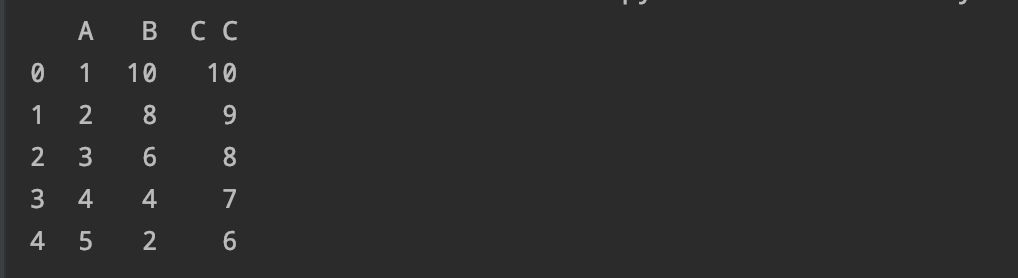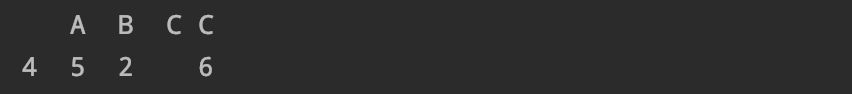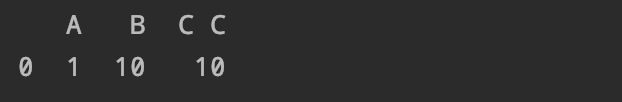1.筛选出指定行之传统方法

df = pd.DataFrame({'A': range(1, 6),

... 'B': range(10, 0, -2),

... 'C C': range(10, 5, -1)})df.A > df.B

df[df.A > df.B]2.筛选出指定行之Query

df.query('A>B')

3.有空格的列名怎么办

df.query('B == `C C`')